4.1 Analysis of Algorithms

# 4.1 Analysis of Algorithms

It is important to pay attention to the cost of the programs that we compose. To study the cost of running our programs, we study them via the scientific method, the commonly accepted body of techniques universally used by scientists to develop knowledge about the natural world. The following five-step approach briefly summarizes the scientific method:

• Observe some feature of the natural world.
• Hypothesize a model that is consistent with the observations.
• Predict events using the hypothesis.
• Verify the predictions by making further observations.
• Validate by repeating until the hypothesis and observations agree.

We also apply mathematical analysis to derive concise models of the cost. In most situations, we are interested in one fundamental characteristic: time.

## Observations

Our first challenge is to make quantitative measurements of the running time of our programs. There are a number of tools available to help us obtain approximations. Perhaps the simplest is a physical stopwatch or the `Stopwatch` data type defined in stopwatch.py (from Section 3.2). We can simply run a program on various inputs, measuring the amount of time to process each input.

With most programs there is a problem size that characterizes the difficulty of the computational task. Normally, the problem size is either the size of the input or the value of a command-line argument. Intuitively, the running time should increase with the problem size, but the question of how much it increases naturally arises every time we develop and run a program.

As a concrete example, we start with threesum.py, which counts the number of triples that sum to 0 in an array of n numbers. Try running it on the files 8ints.txt, 1kints.txt, 2kints.txt, 4kints.txt, 8kints.txt, 16kints.txt, 32kints.txt, 64kints.txt, and 128kints.txt to get a sense of the running time of the program. What is the relationship between the problem size n and the running time for threesum.py?

## Hypotheses

Every programmer needs to know how to make back-of-the-envelope performance estimates. Fortunately, we can often acquire such knowledge by using a combination of empirical observations and a small set of mathematical tools.

### Doubling hypotheses.

For a great many programs, we can quickly formulate a hypothesis for the following question: What is the effect on the running time of doubling the size of the input? For clarity, we refer to this hypothesis as a doubling hypothesis.

### Empirical analysis.

Clearly, we can get a headstart on developing a doubling hypothesis by doubling the size of the input and observing the effect on the running time. For example, doublingtest.py generates a sequence of random input arrays for threesum.py, doubling the array length at each step, and writes the ratio of running times of `threesum.countTriples()` for each input over the previous (which was one-half the size). `Stopwatch` measurements that the program writes lead immediately to the hypothesis that the running time increases by a factor of 8 when the input size doubles.

### Mathematical analysis.

The total running time of a program is determined by two primary factors:

• The cost of executing each statement
• The frequency of execution of each statement

The former is a property of the system, and the latter is a property of the algorithm. If we know both for all instructions in the program, we can multiply them together and sum for all instructions in the program to get the running time.

The primary challenge is to determine the frequency of execution of the statements. Some statements are easy to analyze; for example, the statement that initializes `count` to 0 in `threesum.countTriples()` is executed only once. Others require higher-level reasoning; for example, the `if` statement in `threesum.countTriples()` is executed precisely n(n-1)(n-2)/6 times (that is precisely the number of ways to pick three different numbers from the input array.

To substantially simplify matters in the mathematical analysis, we develop simpler approximate expressions in two ways. First, we work with the leading term of mathematical expressions by using a mathematical device known as the tilde notation. We write ~f(n) to represent any quantity that, when divided by f(n), approaches 1 as n grows. We also write g(n) ~ f(n) to indicate that g(n)/f(n) approaches 1 as n grows. With this notation, we can ignore complicated parts of an expression that represent small values. For example, the `if` statement in `countTriples()` in threesum.py is executed ~n3/6 times because n(n-1)(n-2)/6 = n3/6 - n2/2 + n/3, which, when divided by n3/6, approaches 1 as n grows. This notation is useful when the terms after the leading term are relatively insignificant (for example, when n = 1,000, this assumption amounts to saying that -n2/2 + n/3 ≈ -499,667 is relatively insignificant by comparison with n3/6 ≈ 166,666,667, which it is). Second, we focus on the instructions that are executed most frequently, sometimes referred to as the inner loop of the program. In this program it is reasonable to assume that the time devoted to the instructions outside the inner loop is relatively insignificant.

The key point in analyzing the running time of a program is this: for a great many programs, the running time satisfies the relationship

 T(n) ~ cf(n)

where c is a constant and f(n) is a function known as the order of growth of the running time. For typical programs, f(n) is a function such as log n, n, n log n, n2, or n3 (customarily, we express order-of-growth functions without any constant coefficient). When f(n) is a power of n, as is often the case, this assumption is equivalent to saying that the running time satisfies a power law. In the case of threesum.py, it is a hypothesis already verified by our empirical observations: the order of growth of the running time of threesum.py is n3. The value of the constant c depends both on the cost of executing instructions and on details of the frequency analysis, but we normally do not need to work out the value.

The order of growth is a simple but powerful model of running time. For example, knowing the order of growth typically leads immediately to a doubling hypothesis. In the case of threesum.py, knowing that the order of growth is n3 tells us to expect the running time to increase by a factor of 8 when we double the size of the problem because

 T(2n) / T(n) ~ c(2n)3 / (cn3) = 8

This matches the value resulting from the empirical analysis, thus validating both the model and the experiments.

## Order of Growth Classifications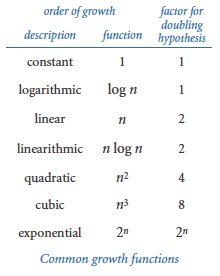We use just a few structural primitives (statements, conditionals, loops, and function calls) to build Python programs, so very often the order of growth of our programs is one of just a few functions of the problem size, summarized in the table at the right.

### Constant.

A program whose running time's order of growth is constant executes a fixed number of statements to finish its job; consequently, its running time does not depend on the problem size. Our first several programs in Chapter 1 — such as helloworld.py (from Section 1.1) and leapyear.py (from Section 1.2) — fall into this category: they each execute several statements just once.

All of Python's operations on standard numeric types take constant time. That is, applying an operation to a large number consumes no more time than does applying it to a small number. (One exception is that operations involving integers with a huge number of digits can consume more than constant time; see the Q & A at the end of this section for details.) The functions in Python's `math` module also take constant time.

### Logarithmic.

A program whose running time's order of growth is logarithmic is barely slower than a constant-time program. The classic example of a program whose running time is logarithmic in the problem size is looking for an element in a sorted array (see binarysearch.py from Section 4.2). The base of the logarithm is not relevant with respect to the order of growth (since all logarithms with a constant base are related by a constant factor), so we usually use log n when referring to the order of growth.

### Linear.

We use the term linear to decribe the order of growth of a program that spends a constant amount of time processing each piece of input data, or that is based on a single `for` loop. The running time of such a program is directly proportional to the problem size. The program average.py (from Section 1.5), which computes the average of the numbers on standard input, is prototypical.

### Linearithmic.

We use the term linearithmic to describe programs whose running time for a problem of size n has order of growth n log n. Again, the base of the logarithm is not relevant. For example, couponcollector.py (from Section 1.4) is linearithmic. The prototypical example is mergesort, as implemented in merge.py (from Section 4.2).

A typical program whose running time has order of growth n2 has two nested `for` loops, used for some calculation involving all pairs of n elements, is said to have quadratic order of growth. The force update double loop in universe.py (from Section 3.4) is a prototype of the programs in this classification, as is the elementary sorting algorithm insertion sort, as defined in insertion.py (see Section 4.2).

### Cubic.

Our example for this section, threesum.py, is cubic — its running time has order of growth n3 — because it has three nested `for` loops, to process all triples of n elements.

### Exponential.

As discussed in Section 2.3, both towersofhanoi.py and beckett.py have running times proportional to 2n because they process all subsets of n elements. Generally, we use the term exponential to refer to algorithms whose order of growth is bn for any constant b > 1, even though different values of b lead to vastly different running times. Exponential algorithms are extremely slow — you should never run one of them for a large problem.

## Python Lists and Arrays

Python's built-in `list` data type represents a mutable sequence of objects. We have been using Python lists throughout the book — recall that we use Python lists as arrays because they support the four core array operations: creation, indexed access, indexed assignment, and iteration. However, Python lists are more general than arrays because you can also insert items into and delete items from Python lists. Even though Python programmers typically do not distinguish between lists and arrays, many other programmers do make such a distinction. For example, in many programming languages, arrays are of fixed length and do not support insertions or deletions. Indeed, all of the array-processing code that we have considered in this book so far could have been done using fixed-length arrays.

The table below gives the most commonly used operations for Python lists.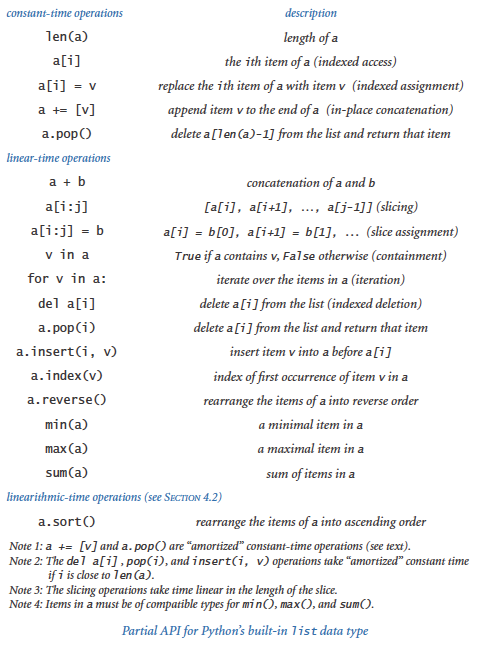We have deferred this API to this section because programmers who use Python lists without paying attention to the cost are in for trouble. For example, consider these two code snippets:

 ```# quadratic time # linear time a = [] a = [] for i in range(n): for i in range(n): a.insert(0, 'slow') a.insert(i, 'fast') ```

The one on the left takes quadratic time; the one on the right takes linear time. To understand why Python list operations have the performance characteristics that they do, you need to learn more about Python's resizing array representation of lists, which we discuss next.

### Resizing arrays.

A resizing array is a data structure that stores a sequence of items (not necessarily fixed in length), which can be accessed by indexing. To implement a resizing array (at the machine level), Python uses a fixed-length array (allocated as one contiguous block of memory) to store the item references. The array is divided into two logical parts: the first part of the array contains the items in the sequence; the second part of the array is unused and reserved for subsequent insertions. Thus, we can append or remove items from the end in constant time, using the reserved space. We use the term size to refer to the number of items in the data structure and the term capacity to refer to the length of the underlying array.

The main challenge is ensuring that the data structure has sufficient capacity to hold all of the items, but is not so large as to waste an excessive amount of memory. Achieving these two goals turns out to be remarkably easy.

First, if we want to append an item to the end of a resizing array, we check its capacity. If there is room, we simply insert the new item at the end. If not, we double its capacity by creating a new array of twice the length and copying the items from the old array into the new array.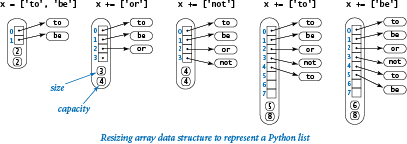Similarly, if we want to remove an item from the end of the resizing array, we check its capacity. If it is excessively large, we halve its capacity by creating a new array of half the length and copying the items from the old array into the new array. An appropriate test is to check whether the size of the resizing array is less than one-fourth of its capacity. That way, after the capacity is halved, the resizing array is about half full and can accommodate a substantial number of insertions before we have to change its capacity again.

The doubling-and-halving strategy guarantees that the resizing array remains between 25% and 100% full, so that space is linear in the number of items. The specific strategy is not sacrosanct. For example, typical Python implementations expand the capacity by a factor of 9/8 (instead of 2) when the resizing array is full. This wastes less space (but triggers more expansion and shrinking operations).

### Amortized analysis.

We can prove that the cost of doubling and halving is always absorbed (to within a constant factor) in the cost of other Python list operations.

Starting from an empty Python list, any sequence of n operations labeled as "constant time" in the list API table shown above takes time linear in n. In other words, the total cost of any such sequence of Python list operations divided by the number of operations is bounded by a constant. This kind of analysis is known as amortized analysis. This guarantee is not as strong as saying that each operation is constant-time, but it has the same implications in many applications (for example, when our primary interest is in total running time).

For the special case where we perform a sequence of n insertions into an empty resizing array, the idea is simple: each insertion takes constant time to add the item; each insertion that triggers a resizing (when the current size is a power of 2) takes additional time proportional to n to copy the elements from the old array of length n to a new array of length 2n. Thus, assuming n is a power of 2 for simplicity, the total cost is proportional to

 (1 + 1 + 1 + ... + 1) + (1 + 2 + 4 + 8 + ... + n) ~ 3n

The first term (which sums to n) accounts for the n insertion operations; the second term (which sums to 2n - 1) accounts for the lg n resizing operations.

Understanding resizing arrays is important in Python programming. For example, it explains why creating a Python list of n items by repeatedly appending items to the end takes time proportional to n (and why creating a list of n items by repeatedly prepending items to the front takes time proportional to n2).

## Strings

Python's string data type has some similarity to Python lists, with one very important exception: strings are immutable. For example, you might think that you could capitalize a string s having the value `'hello'` with `s = 'H'`, but that will result in this run-time error:

 ```TypeError: 'str' object does not support item assignment ```

If you want `'Hello'`, you need to create a completely new string. This difference reinforces the idea of immutability and has significant implications with regard to performance, which we now examine.

### Internal representation.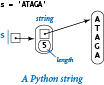First, Python uses a much simpler internal right for strings than for lists/arrays, as detailed in the diagram at right. Specifically, a string object contains two pieces of information:

• A reference to a place in memory where the characters in the string are stored contiguously
• The length of the string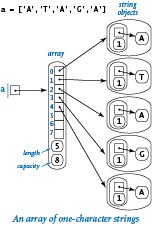By contrast, consider the diagram at left, which is an array of one-character strings. We will consider a more detailed analysis later in this section, but you can see that the string representation is certainly significantly simpler. It uses much less space per character and provides faster access to each character. In many applications, these characteristics are very important because strings can be very long. So, it is important both that the memory usage be not much more than is required for the characters themselves and that characters can be quickly accessed by their index, as in an array.

### Performance.As for arrays, indexed access and computing the length of strings are constant-time operations. It is clear from the API at the beginning of Section 3.1 that most other operations take linear time as a function of the length of the input string or strings, because they refer to a copy of the string. In particular, concatenating a character to a string takes linear time and concatenating two strings takes time proportional to the length of the result. An example is shown at right. With respect to performance, this is the most significant difference between strings and lists/arrays: Python does not have resizable strings, because strings are immutable.

### Example.

Not understanding the performance of string concatenation often leads to performance bugs. The most common performance bug is building up a long string one character at a time. For example, consider the following code fragment to create a new string whose characters are in reverse order of the characters in a string `s`:

 ```n = len(s) reverse = '' for i in range(n): reverse = s[i] + reverse ```

During iteration i of the `for` loop, the string concatenation operator produces a string of length i+1. Thus, the overall running time is proportional to 1 + 2 + ... + n ~ n2 / 2. That is, the code fragment takes quadratic time as a function of the string length n

## Memory

As with running time, a program's memory usage connects directly to the physical world: a substantial amount of your computer's circuitry enables your program to store values and later retrieve them. The more values you need to store at any given instant, the more circuitry you need. To pay attention to the cost, you need to be aware of memory usage.

Python does not define the sizes of the built-in data types that we have been using (`int`, `float`, `bool`, `str`, and `list`); the sizes of objects of those types differ from system to system. Accordingly, the sizes of data types that you create also will differ from system to system because they are based on these built-in data types. The function call `sys.getsizeof(x)` returns the number of bytes that a built-in object `x` consumes on your system. The numbers that we give in this section are observations gathered by using this function in interactive Python on one typical system.

### Integers.

To represent an `int` object whose value is in the range (-263 to 263-1), Python uses 16 bytes for overhead and 8 bytes (that is, 64 bits) for the numeric value. Python switches to a different internal representation for integers outside this range, which consumes memory proportional to the number of digits in the integer, as in the case with strings (see below).

### Floats.

To represent a `float` object, Python uses 16 bytes for overhead and 8 bytes for the numeric value (that is, the mantissa, exponent, and sign), no matter what value the object has. So a float object always consumes 24 bytes.

### Booleans.

In principle, Python could represent a boolean value using a single bit of computer memory. In practice, Python represents boolean values as integers. Specifically, Python uses 24 bytes to represent the `bool` object `True` and 24 bytes to represent the `bool` object `False`. That is a factor of 192 higher than the minimum amount needed! However, this wastefulness is partially mitigated because Python "caches" the two boolean objects.

### Caching.

To save memory, Python creates only one copy of objects with certain values. For example, Python creates only one `bool` object with value true and only one with value false. That is, every boolean variables holds a reference to one of these two objects. This caching technique is possible because the `bool` data type is immutable. On typical systems, Python also caches small `int` values (between -5 and 256), as they are the ones that programmers use most often. Python does not typically cache `float` objects.

### Strings.

To represent a `str` object, Python uses 40 bytes for overhead (including the string length), plus one byte for each character of the string. So, for example, Python represents the string `'abc'` using 40 + 3 = 43 bytes and represents the string `'abcdefghijklmnopqr'` using 40 + 18 = 58 bytes. Python typically caches only string literals and one-character strings.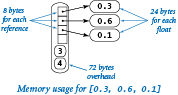### Arrays (Python lists).

To represent an array, Python uses 72 bytes for overhead (including the array length) plus 8 bytes for each object reference (one for each element in the array). So, for example, the Python representation of the array [0.3, 0.6, 0.1] uses 72 + 8*3 = 96 bytes. This does not include the memory for the objects that the array references, so the total memory consumption for the array [0.3, 0.6, 0.1] is 96 + 3*24 = 168 bytes. In general, the memory consumption for an array of n integers or floats is 72 + 32n bytes. This total is likely to be an underestimate, because the resizing array data structure that Python uses to implement arrays may consume an additional n bytes in reserved space.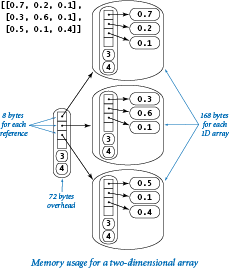### Two-dimensional arrays and arrays of objects.

A two-dimensional array is an array of arrays, so we can calculate the memory consumption of a two-dimensional array with m rows and n columns from the information in the previous paragraph. Each row is an array that consumes 72 + 32n bytes, so the total is 72 (overhead) plus 8m (references to the rows) plus m(72 + 32n) (memory for the m rows) bytes, for a grand total of 72 + 80m + 32mn bytes. The same logic works for an array of any type of object: if an object uses x bytes, an array of m such objects consumes a total of 72 + m(x+8) bytes. Again, this is likely to be a slight underestimate because of the resizing array data structure Python uses to represent arrays. Note: Python's `sys.getsizeof(x)` is not much help in these calculations because it does not calculate the memory for the objects themselves — it returns 72 + 8m for any array of length m (or any two-dimensional array with m rows).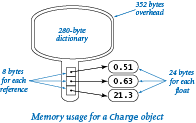### Objects.

A key question for Python programming is the following: How much memory is required to represent a user-defined object? The answer to this question may surprise you, but is important to know: hundreds of bytes, at least. Specifically, Python uses 72 bytes of overhead plus 280 bytes for a dictionary that binds instance variables to objects (we will discuss dictionaries in Section 4.4) plus 24 bytes for a reference to each instance variable plus memory for the instance variables themselves. For example, to represent a `Charge` object, Python uses at least 72 + 280 = 352 bytes for overhead, 8 * 3 = 24 bytes to store the object references for the three instance variables, 24 bytes to store the `float` object referenced by the `_rx` instance variable, 24 bytes to store the `float` object referenced by the `_ry` instance variable, and 24 bytes to store the `float` object referenced by the `_q` instance variable, for a grand total of (at least) 448 bytes. The total might be even higher on your system, because some implementations consume even more overhead.

It is important for every Python programmer to understand that each object of a user-defined type is likely to consume a large amount of memory. So, a Python program that defines a large number of objects of a user-defined type can use much more space (and time) than you might expect. Numerous object-oriented languages have come and gone since the concept was introduced decades ago, and many of them eventually embraced lightweight objects for user-defined types. Python offers two advanced features for this purpose — named tuples and slots — but we will not take advantage of such memory optimizations in this booksite.

#### Q & A

Q. The text notes that operations on very large integers can consume more than constant time. Can you be more precise?

A. Not really. The definition of "very large" is system dependent. For most practical purposes, you can consider operations applied to 32- or 64-bit integers to work in constant time. Modern applications in cryptography involve huge numbers with hundreds or thousands of digits.

Q. How do I find out how long it takes to add or multiply two floats on my computer?

A. Run some experiments! The program timeops.py uses `Stopwatch`, as defined in stopwatch.py from Section 3.2, to test the execution time of various arithmetic operations on integers and floats. This technique measures the actual elapsed time as would be observed on a wall clock. If your system is not running many other applications, it can produce accurate results. Python also includes the `timeit` module for measuring the running time of small code fragments.

Q. Is there any way to measure processor time instead of wall clock time?

A. On some systems, the function call `time.clock()` returns the current processor time as a float, expressed in seconds. When available, you should substitute `time.time()` with `time.clock()` for benchmarking Python programs.

Q. How much time do functions such as `math.sqrt()`, `math.log()`, and `math.sin()` take?

A. Run some experiments! Stopwatch, as defined in stopwatch.py makes it easy to compose programs such as timeops.py to answer questions of this sort for yourself. You will be able to use your computer much more effectively if you get in the habit of doing so.

Q. Why does allocating an array (Python list) of size n take time proportional to n?

A. Python initializes all array elements to whatever values the programmer specifies. That is, in Python there is no way to allocate memory for an array without also assigning an object reference to each element of the array. Assigning object references to each element of an array of size n takes time proportional to n.

Q. How do I find out how much memory is available for my Python programs?

A. Since Python will raise a `MemoryError` when it runs out of memory, it is not difficult to run some experiments. For example, use bigarray.py. Run it like this:

 ```% python bigarray.py 100000000 finished ```

to show that you have room for 100 million integers. But if you type

 ```% python bigarray.py 1000000000 ```

Python will hang, crash, or raise a run-time error; you can conclude that you do not have room for an array of 1 billion integers.

Q. What does it mean when someone says that the worst-case running time of an algorithm is O(n2)?

A. That is an example of a notation known as big-O notation. We write f(n) is O(g(n)) if there exist constants c and n0 such that f(n) ≤ c g(n) for all n > n0. In other words, the function f(n) is bounded above by g(n), up to constant factors and for sufficiently large values of n. For example, the function 30n2 + 10n + 7 is O(n2).We say that the worst-case running time of an algorithm is O(g(n)) if the running time as a function of the input size n is O(g(n)) for all possible inputs. This notation is widely used by theoretical computer scientists to prove theorems about algorithms, so you are sure to see it if you take a course in algorithms and data structures. It provides a worst-case performance guarantee.

Q. So can I use the fact that the worst-case running time of an algorithm is O(n3) or O(n2) to predict performance?

A. No, because the actual running time might be much less. For example, the function 30n2 + 10n + 7 is O(n2), but it is also O(n3) and O(n10) because big-O notation provides only an upper bound on the worst-case running time. Moreover, even if there is some family of inputs for which the running time is proportional to the given function, perhaps these inputs are not encountered in practice. Consequently, you cannot use big-O notation to predict performance. The tilde notation and order-of-growth classifications that we use are more precise than big-O notation because they provide matching upper and lower bounds on the growth of the function. Many programmers incorrectly use big-O notation to indicate matching upper and lower bounds.

Q. How much memory does Python typically use to store a tuple of n items?

A. 56 + 8n bytes, plus whatever memory is needed for the objects themselves. This is a bit less than for arrays because Python can implement a tuple (at the machine level) using an array instead of a resizing array.

Q. Why does Python use so much memory (280 bytes) to store a dictionary that maps an object's instance variables to its values?

A. In principle, different objects from the same data type can have different instance variables. In this case, Python would need some way to manage an arbitrary number of possible instance variables for each object. But most Python code does not call for this (and, as a matter of style, we never need it in this booksite).

#### Exercises

1. Modify threesum.py to take a command-line argument `x` and find a triple of numbers on standard input whose sum is closest to `x`.

2. Compose a program `foursum.py` that takes an integer `n` from standard input, then reads `n` integers from standard input, and counts the number of 4-tuples that sum to zero. Use a quadruple loop. What is the order of growth of the running time of your program? Estimate the largest `n` that your program can handle in an hour. Then, run your program to validate your hypothesis.

3. Prove that 1 + 2 + ... + n = n(n+1)/2.

Solution: We proved this by induction at the beginning of Section 2.3. Here is the basis for another proof:
 ``` 1 + 2 + ... + n-1 + n + n + n-1 + ... + 2 + 1 ---------------------------- n+1 + n+1 + ... + n+1 + n+1 ```
4. Prove by induction that the number of distinct triples of integers between 0 and n-1 is n(n-1)(n-2)/6.

Solution: The formula is correct for n = 2. For n > 2, count all the triples that do not include n-1, which is (n-1)(n-2)(n-3)/6 by the inductive hypothesis, and all the triples that do include n-1, which is (n-1)(n-2)/2, to get the total

 (n-1)(n-2)(n-3)/6 + (n-1)(n-2)/2 = n(n-1)(n-2)/6
5. Show by approximating with integrals that the number of distinct triples of integers between 0 and n-1 is about n3/6.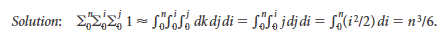6. What is the value of `x` (as a function of n) after running the following code fragment?

 ```x = 0 for i in range(n): for j in range(i+1, n): for k in range(j+1, n): x += 1 ```

Solution: n(n-1)(n-2)/6.

7. Use tilde notation to simplify each of the following formulas, and give the order of growth of each:

• n(n - 1)(n - 2)(n - 3) / 24
• (n - 2) (lg n - 2) (lg n + 2)
• n(n + 1) - n2
• n(n + 1)/2 + n lg n
• ln((n - 1)(n - 2) (n - 3))2
8. Is the following code fragment linear, quadratic, or cubic (as a function of n)?

 ```for i in range(n): for j in range(n): if i == j: c[i][j] = 1.0 else: c[i][j] = 0.0 ```
9. Suppose the running time of an algorithm on inputs of size 1000, 2000, 3000, and 4000 is 5 seconds, 20 seconds, 45 seconds, and 80 seconds, respectively. Estimate how long it will take to solve a problem of size 5000. Is the algorithm linear, linearithmic, quadratic, cubic, or exponential?

10. Which would you prefer: a quadratic, linearithmic, or linear algorithm?

Solution: While it is tempting to make a quick decision based on the order of growth, it is very easy to be misled by doing so. You need to have some idea of the problem size and of the relative value of the leading coefficients of the running time. For example, suppose that the running times are n2 seconds, 100 n log2 n seconds, and 10,000 n seconds. The quadratic algorithm will be fastest for n up to about 1000, and the linear algorithm will never be faster than the linearithmic one (n would have to be greater than 2100, far too large to bother considering).

11. Apply the scientific method to develop and validate a hypothesis about order of growth of the running time of the following code fragment, as a function of the input argument `n`.

 ```def f(n): if (n == 0): return 1 return f(n-1) + f(n-1) ```
12. Apply the scientific method to develop and validate a hypothesis about order of growth of the running time of each of the following two code fragments as a function of `n`.

 ```s = '' for i in range(n): if stdrandom.bernoulli(0.5): s += '0' else: s += '1' ```
 ```s = '' for i in range(n): oldS = s if stdrandom.bernoulli(0.5): s += '0' else: s += '1' ```

Solution: On many systems, the first is linear; the second is quadratic. You have no way of knowing why: In the first case, Python detects that s is the only variable that refers to the string, so it appends each character to the string as it would with a list (in amortized constant time) even though the string is immutable! A safer alternative is to create a list containing the characters and concatenate them together with by calling the `join()` method.

 ```a = [] for i in range(n): if stdrandom.bernoulli(0.5): a += ['0'] else: a += ['1'] s = ''.join(a) ```
13. Each of the four Python functions below returns a string of length `n` whose characters are all `x`. Determine the order of growth of the running time of each function. Recall that concatenating two strings in Python takes time proportional to the sum of their lengths.

 ```def f1(n): if (n == 0): return '' temp = f1(n // 2) if (n % 2 == 0): return temp + temp else: return temp + temp + 'x' ```
 ```def f2(n): s = '' for i in range(n): s += 'x' return s ```
 ```def f3(n): if (n == 0): return '' if (n == 1): return 'x' return f3(n//2) + f3(n - n//2) ```
 ```def f4(n): temp = stdarray.create1D(n, 'x') return ''.join(temp) ```
 ```def f5(n): return 'x' * n ```
14. The following code fragment (adapted from a Java programming book) creates a random permutation of the integers from 0 to n-1. Determine the order of growth of its running time as a function of n. Compare its order of growth with the shuffling code in Section 1.4.

 ```a = stdarray.create1D(n, 0) taken = stdarray.create1D(n, False) count = 0 while (count < n): r = stdrandom.uniformInt(n) if not taken[r]: a[r] = count taken[r] = True count += 1 ```
15. How many times does the following code fragment execute the first `if` statement in the triply nested loop?

 ```for i in range(n): for j in range(n): for k in range(n): if (i < j) and (j < k): if a[i] + a[j] + a[k] == 0: count += 1 ```

16. Apply the scientific method to develop and validate a hypothesis about order of growth of the running time of the `collect()` method in coupon.py (from Section 2.1), as a function of the argument `n`. Note: Doubling is not effective for distinguishing between the linear and linearithmic hypotheses — you might try squaring the size of the input.

17. Apply the scientific method to develop and validate a hypothesis about order of growth of the running time of markov.py (from Section 1.6), as a function of the arguments `moves` and `n`.

18. Compose a program `mooreslaw.py` that takes a command-line argument n and writes the increase in processor speed over a decade if processor speed doubles every n months. How much will processor speed increase over the next decade if speeds double every n = 15 months? 24 months?

19. Using the memory model from the text, give the memory requirements for each object of the following data types from Chapter 3:

• `Stopwatch`
• `Turtle`
• `Vector`
• `Body`
• `Universe`
20. Estimate, as a function of the grid size n, the amount of space used by visualizev.py (from Section 2.4) with the vertical percolation detection. Extra credit: Answer the same question for the case where the recursive percolation detection method in percolation.py is used.

21. Estimate the size of the largest n-by-n array of integers that your computer can hold, and then try to allocate such an array.

22. Estimate, as a function of the number of documents n and the dimension d, the amount of space used by comparedocuments.py (from Section 3.3).

23. Compose a version of primesieve.py (from Section 1.4) that uses an array of integers instead of an array of booleans and uses 32 bits in each integer, to raise the largest value of n that it can handle by a factor of 32.

24. The following table gives running times for various programs for various values of n. Fill in the blanks with estimates that you think are reasonable on the basis of the information given.

program 1,000 10,000 100,000 1,000,000
A 0.001 seconds 0.012 seconds 0.16 seconds ? seconds
B 1 minute 10 minutes 1.7 hours ? hours
C 1 second 1.7 minutes 2.8 hours ? days

Give hypotheses for the order of growth of the running time of each program.

#### Creative Exercises

1. Three-sum analysis. Calculate the probability that no triple among n random 32-bit integers sums to 0, and give an approximate estimate for n equal to 1000, 2000, and 4000. Extra credit: Give an approximate formula for the expected number of such triples (as a function of n), and run experiments to validate your estimate.

2. Closest pair. Design a quadratic algorithm that finds the pair of integers that are closest to each other. (In the next section you will be asked to find a linearithmic algorithm.)

3. Power law. Show that a log-log plot of the function cnb has slope b and x-intercept log c. What are the slope and x-intercept for 4 n3(log n)2?

4. Sum furthest from zero. Design an algorithm that finds the pair of integers whose sum is furthest from zero. Can you discover a linear algorithm?

5. The "beck" exploit. A popular web server supports a function called `no2slash()` whose purpose is to collapse multiple `/` characters. For example, the string `/d1///d2////d3/test.html` becomes `/d1/d2/d3/test.html`. The original algorithm was to repeatedly search for a `/` and copy the remainder of the string:

 ```def no2slash(name): nameList = list(name) x = 1 while x < len(nameList): if (nameList[x-1] == '/') and (nameList[x] == '/'): for y in range(x+1, len(nameList)): nameList[y-1] = nameList[y] nameList = nameList[:-1] else: x += 1 return ''.join(nameList) ```

Unfortunately, the running time of this code is quadratic in the number of `/` characters in the input. By sending multiple simultaneous requests with large numbers of `/` characters, a hacker can deluge a server and starve other processes for CPU time, thereby creating a denial-of-service attack. Develop a version of `no2slash()` that runs in linear time and does not allow for the this type of attack.

6. Young tableaux. Suppose you have in memory an n-by-n grid of integers `a[][]` such that `a[i][j] < a[i+1][j]` and `a[i][j] < a[i][j+1]` for all `i` and `j`, like the table below.

 ``` 5 23 54 67 89 6 69 73 74 90 10 71 83 84 91 60 73 84 86 92 90 91 92 93 94 ```

Devise an algorithm whose order of growth is linear in n to determine whether a given integer x is in a given Young tableaux.

Solution: Start at the upper-right corner. If the value is x, return `True`. Otherwise, go left if the value is greater than x and go down if the value is less than x. If you reach bottom left corner, then x is not in table. The algorithm is linear because you can go left at most n times and down at most n times.

7. Subset sum. Compose a program `anysum.py` that takes an integer n from standard input, then reads n integers from standard input, and counts the number of subsets that sum to 0. Give the order of growth of the running time of your program.

8. Array rotation. Given an array of n elements, give a linear time algorithm to rotate the array k positions. That is, if the array contains a0, a1, ..., an-1, the rotated array is ak, ak+1, ..., an-1, a0, ..., ak-1. Use at most a constant amount of extra space (array indices and array values). Hint: Reverse three subarrays.

9. Finding a duplicated integer. (a) Given an array of n integers from 1 to n with one value repeated twice and one missing, give an algorithm that finds the missing integer, in linear time and constant extra space. (b) Given a read-only array of n integers, where each value from 1 to n-1 occurs once and one occurs twice, give an algorithm that finds the duplicated value, in linear time and constant extra space. (c) Given a read-only array of n integers with values between 1 and n-1, give an algorithm that finds a duplicated value, in linear time and constant extra space.

10. Factorial. Design a fast algorithm to compute n! for large values of n. Use your program to compute the longest run of consecutive 9s in 1000000!. Develop and validate a hypothesis for the order of growth of the running time of your algorithm.

11. Maximum sum. Design a linear algorithm that finds a contiguous subsequence of at most m in a sequence of n integers that has the highest sum among all such subsequences. Implement your algorithm, and confirm that the order of growth of its running time is linear.

12. Pattern matching. Given an n-by-n array of black (1) and white (0) pixels, design a linear algorithm that finds the largest square subarray that consists of entirely black pixels. As an example, the following 8-by-8 array contains a 3-by-3 subarray entirely of black pixels.

 ```1 0 1 1 1 0 0 0 0 0 0 1 0 1 0 0 0 0 1 1 1 0 0 0 0 0 1 1 1 0 1 0 0 0 1 1 1 1 1 1 0 1 0 1 1 1 1 0 0 1 0 1 1 0 1 0 0 0 0 1 1 1 1 0 ```

Implement your algorithm and confirm that the order of growth of its running time is linear in the number of pixels. Extra credit: Design an algorithm to find the largest rectangular black subarray.

13. Maximum average. Compose a program that finds a contiguous subarray of at most m elements in an array of n integers that has the highest average value among all such subarrays, by trying all subarrays. Use the scientific method to confirm that the order of growth of the running time of your program is mn2. Next, compose a program that solves the problem by first computing `prefix[i] = a + ... + a[i]` for each `i`, then computing the average in the interval from `a[i]` to `a[j]` with the expression `(prefix[j] - prefix[i]) / (j - i + 1)`. Use the scientific method to confirm that this method reduces the order of growth by a factor of n.

14. Sub-exponential function. Find a function whose order-of-growth is slower than any polynomial function, but faster than any exponential function. Extra credit: Compose a program whose running time has that order of growth.

15. Resizing arrays. For each of the following strategies, either show that each resizing array operation takes constant amortized time or find a sequence of n operations (starting from an empty data structure) that takes quadratic time.

1. Double the capacity of the resizing array when it is full and halve the capacity when it is half full.
2. Double the capacity of the resizing array when it is full and halve the capacity when it is one-third full.
3. Increase the capacity of the resizing array by a factor of 9/8 when it is full and decrease it by a factor of 9/8 when it is 80% full.